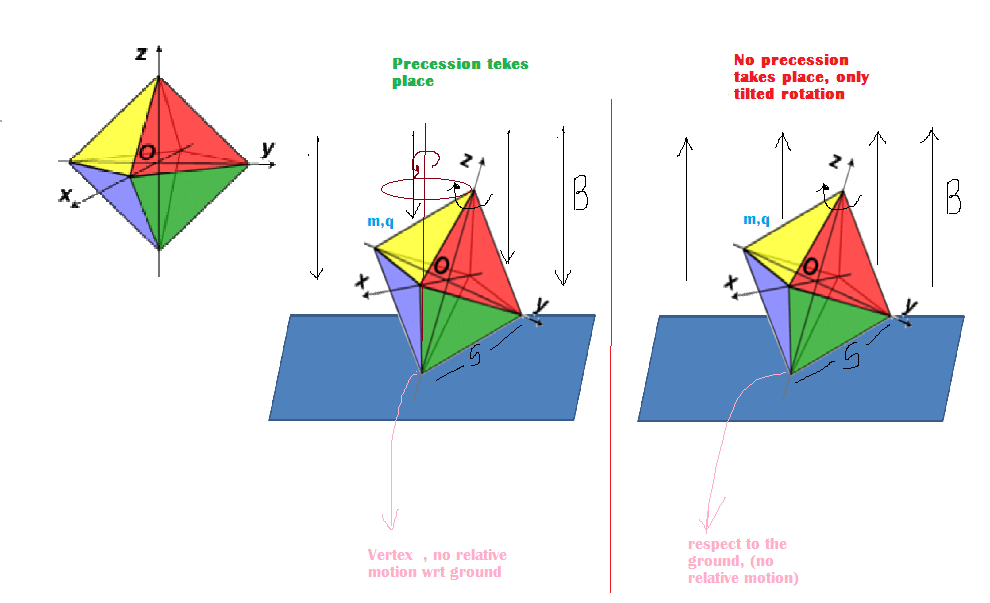# Critical Angular Velocity of Tilted RotationA top is rotated in the presence of a uniform magnetic field (directed vertically downwards)on a rough horizontal surface. The top precesses around a vertical axis . Now the polarity of the magnetic field is reversed and again the top is spun with the same angular velocity but now it is observed that top does not precess but undergoes rotation about which ever axis it was rotated initially. Find the angular velocity of the top.

The top in this case is a hollow octahedron of mass $m$, charge $q$ and side $s$ and the magnetic field has a magnitude $B$ then the critical angular velocity with which the top has to be spun (about an axis passing through two opposite vertices of the octahedron) can be represented as $\omega=a\frac{mg}{qsB}$ then find the units digit of [100a]

Assumptions

• The point of contact i.e. vertex is stationary mass and charge is uniformly distributed along the faces.
• Refer to gyro -magnetic ratio rather that calculating magnetic moment. For a octahedron ratio of square of radius of gyration to the square is side $s$ is $5/9$.
• $\left[x\right]$ represents the greatest integer function.
• In both of the cases the tops are rotating in anti clockwise sense, (not as shown in the figure which is clockwise)
×